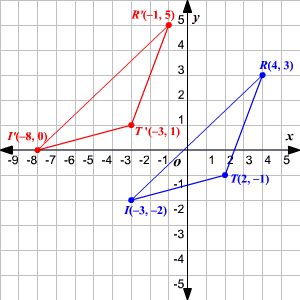# Transformation of Graphs Using Matrices - Translation

A type of transformation that occurs when a figure is moved from one location to another on the coordinate plane without changing its size, shape or orientation is a translation Matrix addition can be used to find the coordinates of the translated figure.

Example:

Find the coordinates of the vertices of triangle $TRI$ with $T\left(2,-1\right),R\left(4,3\right)\text{\hspace{0.17em}}\text{\hspace{0.17em}}\text{and}\text{\hspace{0.17em}}\text{\hspace{0.17em}}I\left(-3,-2\right)$ if it is to be moved $5$ units to the left and $2$ units up.

Write the coordinates of the triangle as a coordinate matrix.

$\left[\begin{array}{ccc}\hfill 2& \hfill 4& \hfill -3\\ \hfill -1& \hfill 3& \hfill 2\end{array}\right]$

Translating the triangle $5$ units to the left means that each $x$ -coordinate decreases by $5$ .

Translating the triangle $2$ units up means that each $y$ -coordinate increases by $2$ .

The translation matrix that will do this is

$\left[\begin{array}{ccc}\hfill -5& \hfill -5& \hfill -5\\ \hfill 2& \hfill 2& \hfill 2\end{array}\right]$

To find the coordinates of the vertices of the translated triangle ${T}^{\text{'}}{R}^{\text{'}}{I}^{\text{'}}$ add the translation matrix to the coordinate matrix:

$\left[\begin{array}{ccc}\hfill 2& \hfill 4& \hfill -3\\ \hfill -1& \hfill 3& \hfill -2\end{array}\right]+\left[\begin{array}{ccc}\hfill -5& \hfill -5& \hfill -5\\ \hfill 2& \hfill 2& \hfill 2\end{array}\right]=\left[\begin{array}{ccc}\hfill -3& \hfill -1& \hfill -8\\ \hfill 1& \hfill 5& \hfill 0\end{array}\right]$

The coordinates of the vertices of ${T}^{\text{'}}{R}^{\text{'}}{I}^{\text{'}}$ are ${T}^{\text{'}}\left(-3,1\right),{R}^{\text{'}}\left(-1,5\right)\text{\hspace{0.17em}}\text{\hspace{0.17em}}\text{and}\text{\hspace{0.17em}}\text{\hspace{0.17em}}{I}^{\text{'}}\left(-8,0\right)$ .